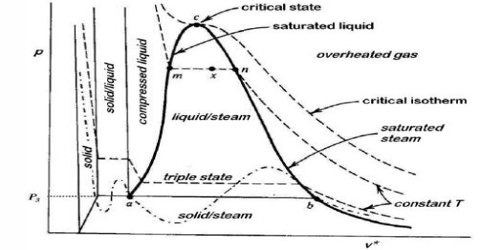Chemistry

# Heike Kamerlingh Onnes Equations of StateHeike Kamerlingh Onnes Equations of State

Many other equations of state have been proposed at different times. None of those were, however, entirely satisfactory; neither did these have better theoretical foundation than the equation of van der Waals and consequently have not found general acceptance. The most general equation relating P, V and T of a gas is that due to the Dutch physicist, Heike Kamerlingh Onnes (1901) and is written as;

PV = RT [1 + BT/V + CT/V2

Where B(T), C(T), etc. which are functions of the temperature, are called the virial co-efficients. This equation is superior in the sense that other equations of state may be derived from this equation by suitable replacement of the values of the virial co-efficients.

This equation is also called the Kamerlingh Onnes equation. If appropriate assumptions are made about the mathematical form of intermolecular forces, theoretical expressions can be developed for each of the coefficients.